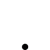# What is E.M.F

• 150 Views
• Last Post 4 weeks ago
Chris posted this 4 weeks ago

My Friends,

I have seen some confusion lately on E.M.F, I want to try to clear this confusion up simple as possible.

Here is the definition of E.M.F:

In electromagnetism and electronics, electromotive force (emf, denoted ε and measured in volts) is the electrical action produced by a non-electrical source. Devices (known as transducers) provide an emf by converting other forms of energy into electrical energy, such as batteries (which convert chemical energy) or generators (which convert mechanical energy). Sometimes an analogy to water pressure is used to describe electromotive force. (The word "force" in this case is not used to mean forces of interaction between bodies).

Faradays Law of Electromagnetic Induction predicts Electromotive Force:

### E.M.F = -N dΦ/dt

Electromotive Force, which, there is actually no Force at all here, it is Charge, or Energy per Unit Charge, not Force!

E.M.F is Measured in Units of Volts, here is the definition of Volts:

Voltage, electric potential difference, electric pressure or electric tension is the difference in electric potential between two points, which (in a static electric field) is defined as the work needed per unit of charge to move a test charge between the two points. In the International System of Units, the derived unit for voltage (potential difference) is named volt. In SI units, work per unit charge is expressed as joules per coulomb, where 1 volt = 1 joule (of work) per 1 coulomb (of charge).

Now, Suppose we have a Circuit, it is active, its operational, running, and we need to calculate the E.M.F, how do we go about this? Well its very simple! Very simple!

Ohms Law gives us the Solution, V = IR and some may like to see the equation slightly rewritten: E = IR;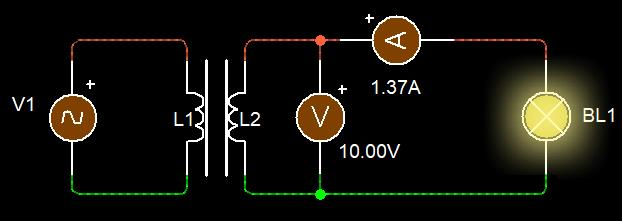In this Circuit, we have two components, a Coil and a Light (Lamp). The Coil has an E.M.F Induced in it via Faradays Law of Electromagnetic Induction. We all know this off by heart by now.

We need to calculate the E.M.F, and this is really simple!

E.M.F ( ε ) = I x ( RCoil + RLamp ) = 1.36986 x ( 0.3 + 7 ) = 10 Volts.

So we see here, we have an Internal Resistance in the Coil, normally Impedance, and the Load, the Light or Lamp has a Resistance also! So we can see, we now have  a voltage that is measured and calculated, as an Open Circuit Voltage.

So, what's the difference between E.M.F and Voltage? Nothing, we Measure the E.M.F as the Open Circuit Voltage, that's all.

Some friends are asking questions about Electromagnetic Induction in the area of: "What keeps the Electrons Moving?" An excellent question to ask!

Answer: Try switching off your Input Current Instantaneously, then see what happens to your Output Current, it falls to Zero immediately also!

We can have E.M.F with No Current, remember this. We can not however have Output Current without Input Current, Input Current is the Supporting and stabilizing factor for Output Current, without this Support, there can be no Output Current.

This experiment can also be done with a heavy duty Potentiometer on the Input, Start at Zero R, then Instantaneously turn it to max, watch your Output Current fall as a result of the Input Circuit Resistance.

NOTE: E.M.F is dependent of the Change, dΦ/dt, where Current is NOT! At least not after the initial Gain in E.M.F. Remember Current I is Dependent on Voltage V, or E.M.F. Input Current can be stable with No Change in Time, and Output Current will also be the same. So once Voltage is stable, Current can also then be Stable, DC Output.

In other words, Bucking Magnetic Fields Pump Current!

I have shown this here: @ 14 : 44

and here @ 25 : 01

This last part IS a Hole in Electromagnetic Induction and NOT Explained! I have just explained it to you and you now have the Answer.

For Reference, this website is a very good read!

So again, what's the difference between E.M.F and Voltage?

Nothing, we Measure the E.M.F as the Open Circuit Voltage.

Best Wishes My Friends,

Chris

alannn posted this 4 weeks ago

Thanks for this post.

EMF is absolute and a characteristic of the transducer only, voltage is relative, a potential difference between two random points.

I have a question too: what makes electrons flow into amps and powers the circuits? The electric field of an emf potential,or the charges that are forced in to the circuits?

Chris posted this 4 weeks ago

Hey Alannn,

I in turn, ask you a question:

What keeps a Marble Rolling on a Flat Surface?

I have a question too: what makes electrons flow into amps and powers the circuits? The electric field of an emf potential,or the charges that are forced in to the circuits?

We must first look at some fundamental Definitions.

One Ampere has recently been redefined as:

1 ampere will be the electric current corresponding to the flow of 1/(1.602 176 634 × 10−19) elementary charges per second.

See Here for the Document.

Ok, now whats the Elementary Charge Defined as:

The elementary charge, usually denoted by e or sometimes qe is the electric charge carried by a single proton or, equivalently, the magnitude of the negative electric charge carried by a single electron, which has charge −1 e.

This elementary charge is a fundamental physical constant. To avoid confusion over its sign, e is sometimes called the elementary positive charge.

From the 2019 redefinition of SI base units, which took effect on 20 May 2019, its value is exactly 1.602176634×10−19 C, by definition of the coulomb. In the centimetre–gram–second system of units (CGS), it is 4.80320425(10)×10−10 statcoulombs.

Calculating this out, the definition of One Ampere, we get a figure of:

One Ampere = 6,241,509,074,460,760,000 elementary charges per second

This is past Point P1 in One Second, because we have Volumetric Flow.

Now we need to look at what the Elementary Charge is! We will focus on the Electron, but all Elementary Charges have the same basic properties: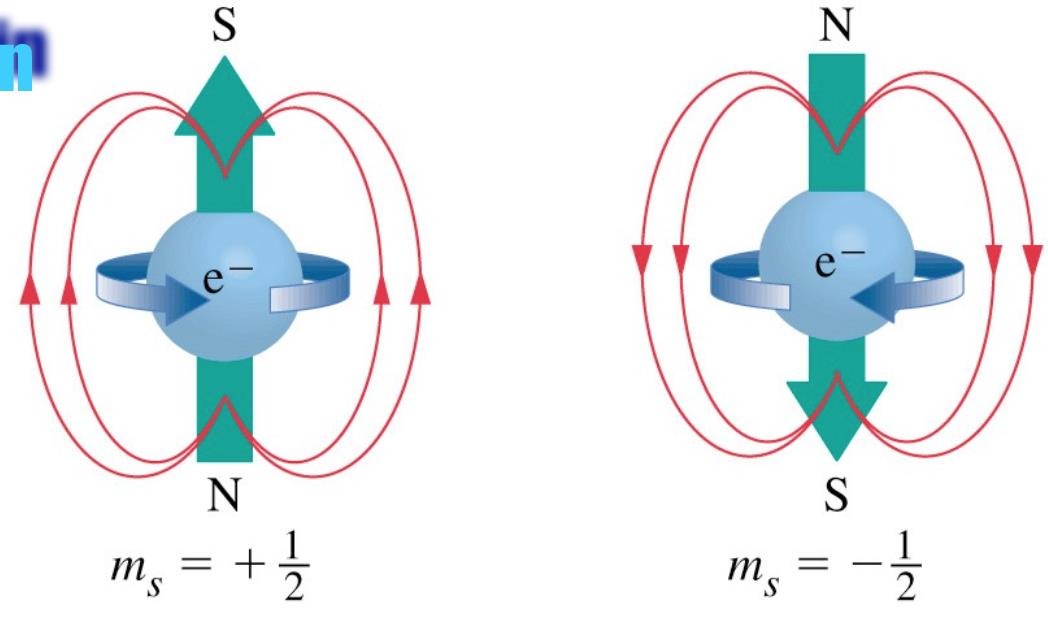All Elementary Charges, because they have a Charge, they also have several other properties:

• Mass
• Magnetic Field known as the Magnetic Moment
• Spin, shown above, as +1/2 or -1/2
• Of course, as stated, they have Charge, the fundamental property.

Floyd Sweet had this to say:

Conventional theory says that electric fields and magnetic fields are different things. Consider for a moment a charge with an electric field around it. If the charge is moved a magnetic field develops and the moving charge constitutes a current. If an observer were to move along with the charge, he would see no relative motion, no current, and no magnetic field. A stationary observer would see motion, current and a magnetic field. It would appear that a magnetic field is an electric field observed from a motional reference frame. Similarly, if we take a mass with a gravity field around it and we move the mass and create a mass current, a new field is also created. It is a different kind of gravity field with no source and no sink.

and then

They are now able to simply support mass, as demonstrated with the transformer steel illustration.

"They" are in reference to the Magnets. It took me some time to understand this, because

Because the Electron has Charge and thus a Magnetic Field, we can minipulate the Chargeed Particle, the Cyclotron does exactly this! We can use an External Magnetic Field, to force the Charged Particle to move, this is the best analogy I have found for this:

Inside the wire, it looks like this:

So what makes the Electrons move, the Magnetic Field of the Primary makes the Electrons in the Secondary Move in a specific Direction. This is explained by The Lorentz Force.

Here is a cool experiment showing what Charge does in a Magnetic Field:

and another:

The last thing, we have Elementary Charge Propagation, a topic very much confused and clouded in Science today!

Antenna Theory shows, with experiment to also prove it, that the Elementary Charge has a Velocity ( v ), and we can very easily calculate this Velocity with two other known factors:

1. Frequency
2. Wavelength

Here is the triangle: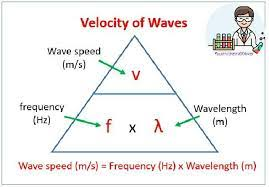Here is a short calculation to show the speed in an Antenna:

At Antenna Resonance, we can calculate the Velocity of the very same Charge Carriers: Electrons, by using the simple math of Antenna Theory: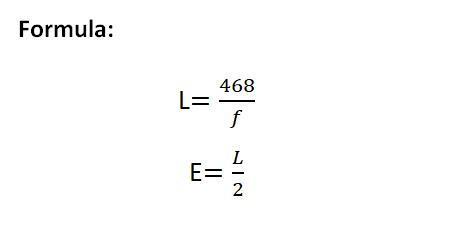Of course, the Velocity is stated as 468, so a 1 Meter Dipole Antenna at 1MHz, we have a length of 468 feet. Or in Meters: 142.65 meters. This is at MHz and Per Second.

Calculating this out, if we use: 299.792458 MHz, we get, Total length of dipole:

• 1 foot, 6.7 inches
• 0.48 meters

Length of each element:

• 0 feet, 9.4 inches
• 0.24 meters

Of course, using Wavelength Equations, we can get Velocity also: v = f x λ = 299792458 x (2 x 0.48 meters) = 287,800,759.68 Meters per second. Slightly slower than the speed of light, 0.96, or 96% of the Speed of Light.

The Mass Movement of Electrons or Charge, defines the Amplitude of an Electromagnetic Wave, they are one and the same things. More Charge/Electrons Moving, higher the Amplitude of the EM Wave. We have seen this in Magnetic Fields for a long time now! Antenna Theory confirms this very fundamental requirement. The definition for the Magnetic Field is Charge in Motion!

Wikipedia admits to this ambiguous definition:

The word electricity refers generally to the movement of electrons (or other charge carriers) through a conductor in the presence of a potential difference or an electric field. The speed of this flow has multiple meanings. In everyday electrical and electronic devices, the signals travel as electromagnetic waves typically at 50%–99% of the speed of light, while the electrons themselves move much more slowly, see Drift velocity and Electron mobility.

I did answer your question in the first post, but wanted to further explain here.

We believe in the systematic understanding of the physical world, through observation and experimentation, through argument and debate. But most of all through freedom of will.

Ref: The Ark of Truth

Ironically, an old 1954 declassified video shows us this:

So, "what makes electrons flow into amps", it is M.M.F, Magnetomotive Force, the Work Component in Electromagnetic Induction, that makes the Electrons Move and keeps moving them. They are under Force, via The Lorentz Force. This is the same as your Finger pushing the Marble on the Flat Surface.

The answers are there, one must look in the right places.

Best Wishes,

Chris

alannn posted this 4 weeks ago

Thanks for the elaborate post! Sorry that it didn't answer my question, because I was thinking about it more elementary, not taking induction into account (yet). Let's state it differently: When we short a battery, what causes the electron flow? The electrostatic force field of the battery voltage potential (transducer EMF), or the pressure of the charges that want to escape into the circuit?

Chris posted this 4 weeks ago

Hi Alannn,

Ah yes got ya, sorry totally missunderstood your question.

Yes, there is a different situation here! Youre talking Outside the "Separation" Process, in the external Circuit... Sort of off topic I think!

I am talking Internal Processes, what's the Processes to allow for an E.M.F. Processes very few EE's are taught and ever think about!

Chemical Charge Separation is the Answer. As a Battery has an Internal Resistance, normally very low, the Separation of Charges from the Chemical Process occuring in the Battery is regulated by this Internal Resistance, both Ohmic Resistance and Ionic Resistance, and we see a limited Current to the Load. This is called Battery Impedance and can be easily measured.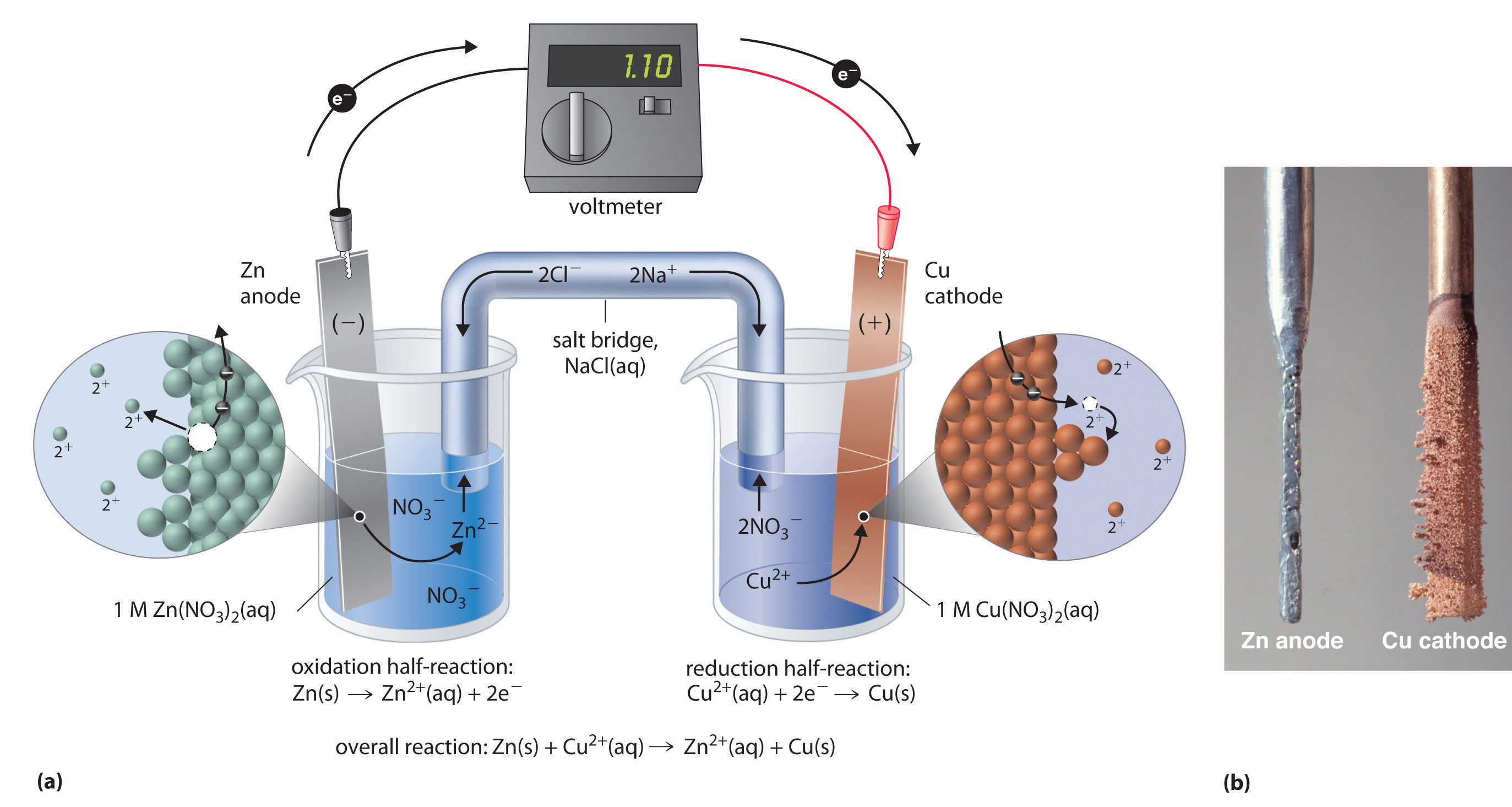As Charge is Separated, there is a need for the distribution of Charge to Ballance out, and you could say there is an Electrostatic Force that acts on the Charges to distribute then evenly, falling back to Equilibrium. Just like Lightning eventually Discharges to a lesser Charged Source.

I have a video I copied and posted here:

However, Electrostatic Force acting upon the Charged Particles is not enough in its own right, to keep Electrons Flowing. So the reason for Current is not Electrostatic Force. There can be no Current with no E.M.F. Current is entirely due to the continuois Separation of Charges always wanting to discharge to the Lesser Charged Terminal.

Yes, from the Battery Terminals, out into the External Circuit, the Separated Charges wants to Distribute itself, Electrostatic Discharge is due to Static Charge build-up, like Lightning.

Which should be covered in another thread, perhaps: Circuit Theory?

This video on Solar Cells explains very well the Separation of Charges:

This is why, the Chemical Process in the Battery, Batterys Heat up under Loads larger than what they are rated for and can be dammaged as a result.

Here is a very good video on batteries:

In saying what I have said, I do believe you know all this anyway by the sounds of what you wrote.

Best Wishes,
Chris

Since Nov 27 2018
Recomended Protocol:Measurement
Our Above Unity Machines:Romanian ZPMWistiti's Bucking Joule ThiefYo's Ferro-Magnetic ResonanceJagau's Non-linear ResonanceCD's Non-Inductive Coil RepNon-Inductive Coil ExperimentBaerndorfer's ExperimentCaptainloz's A-R-EFerrite at work
Start Here:Help with using the Forum.

More than anything else, your contributions to this forum are most important! We are trying to actively get all visitors involved, but we do only have a few main contributors, which are very much appreciated! If you would like to see more pages with more detailed experiments and answers, perhaps a contribution of another type maybe possible:

PayPal De-Platformed me!

They REFUSE to tell me why!

We now use Wise!

Donate

The content I am sharing is not only unique, but is changing the world as we know it! Please Support Us!

Perhaps a Bank Transfer?

• Bank: Bank of Queensland
• Name: Christopher R Sykes
• BSB: 124-001
• Account: 21580359

Thank You So Much!

Weeks High Earners:
The great Nikola Tesla:Ere many generations pass, our machinery will be driven by a power obtainable at any point of the universe. This idea is not novel. Men have been led to it long ago go by instinct or reason. It has been expressed in many ways, and in many places, in the history of old and new. We find it in the delightful myth of Antheus, who drives power from the earth; we find it among the subtle speculations of one of your splendid mathematicians, and in many hints and statements of thinkers of the present time. Throughout space there is energy. Is this energy static or kinetic? If static, our hopes are in vain; if kinetic - and this we know it is for certain - then it is a mere question of time when men will succeed in attaching their machinery to the very wheelwork of nature.

Experiments With Alternate Currents Of High Potential And High Frequency (February 1892).

Close